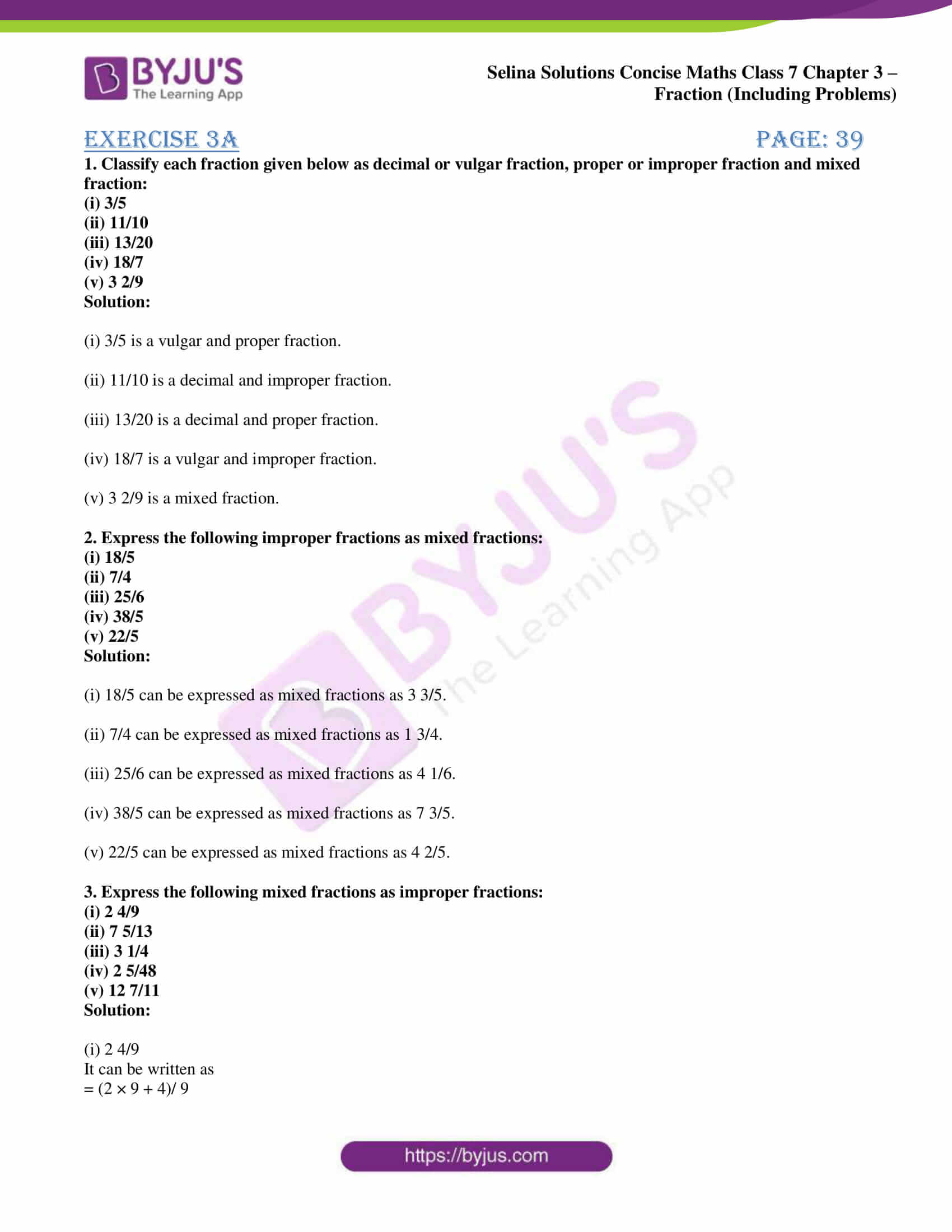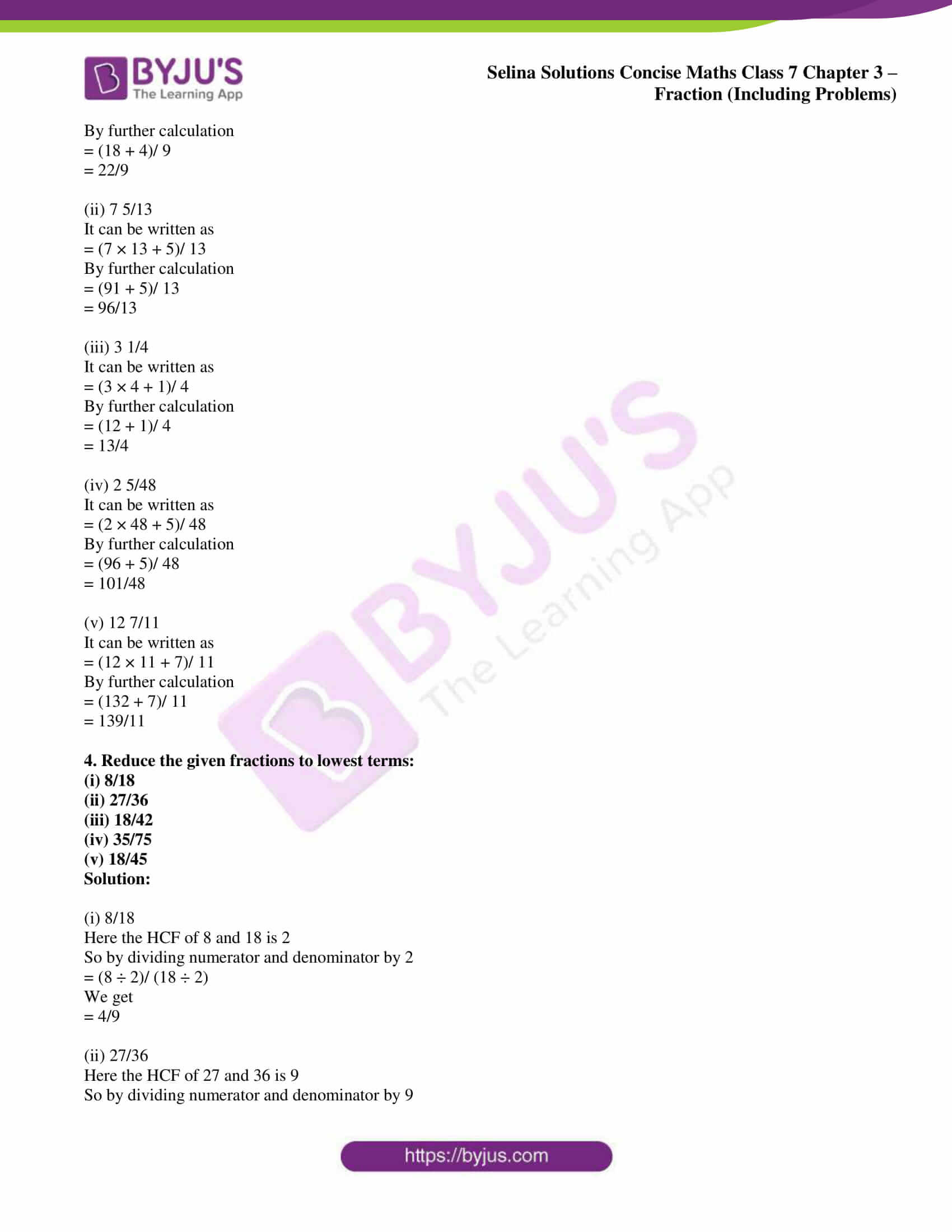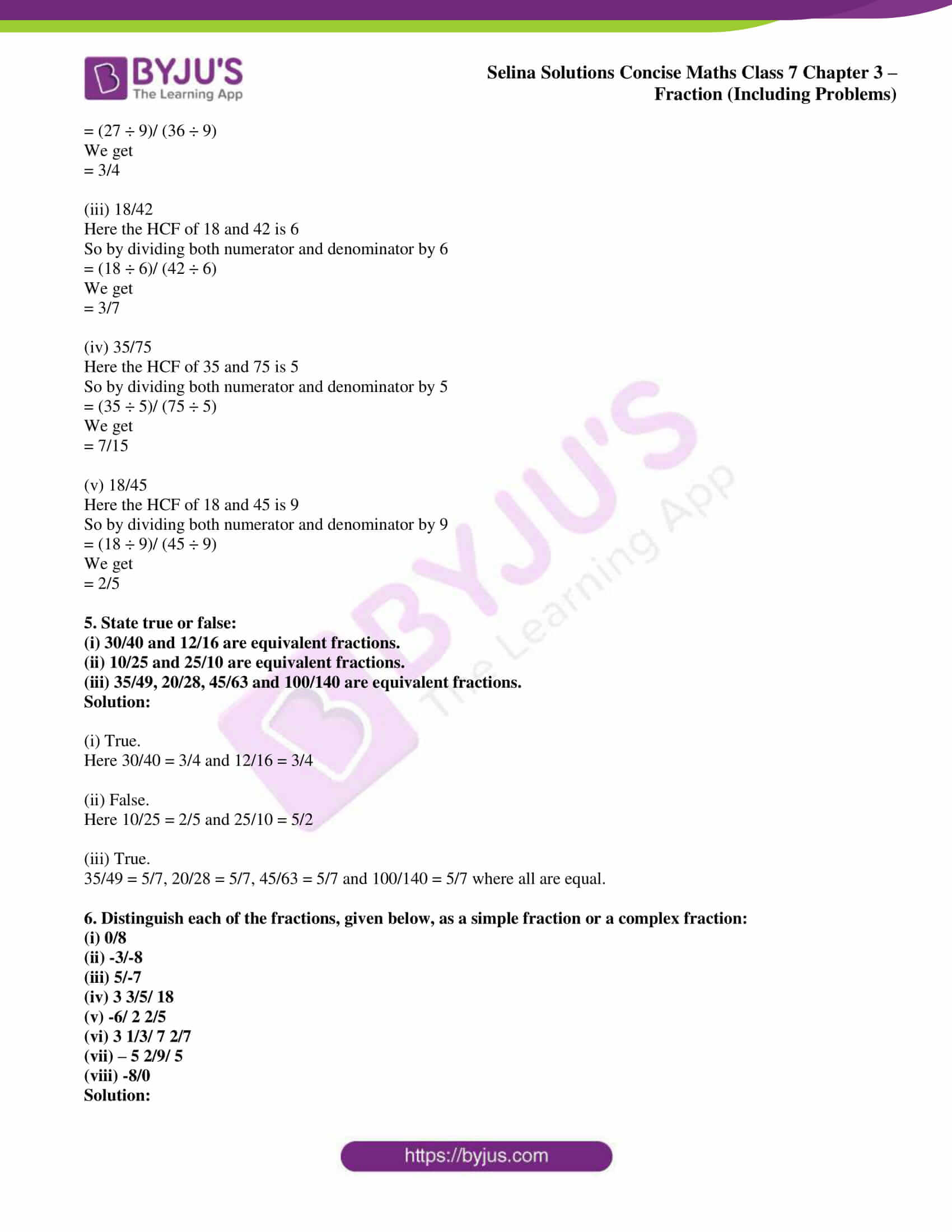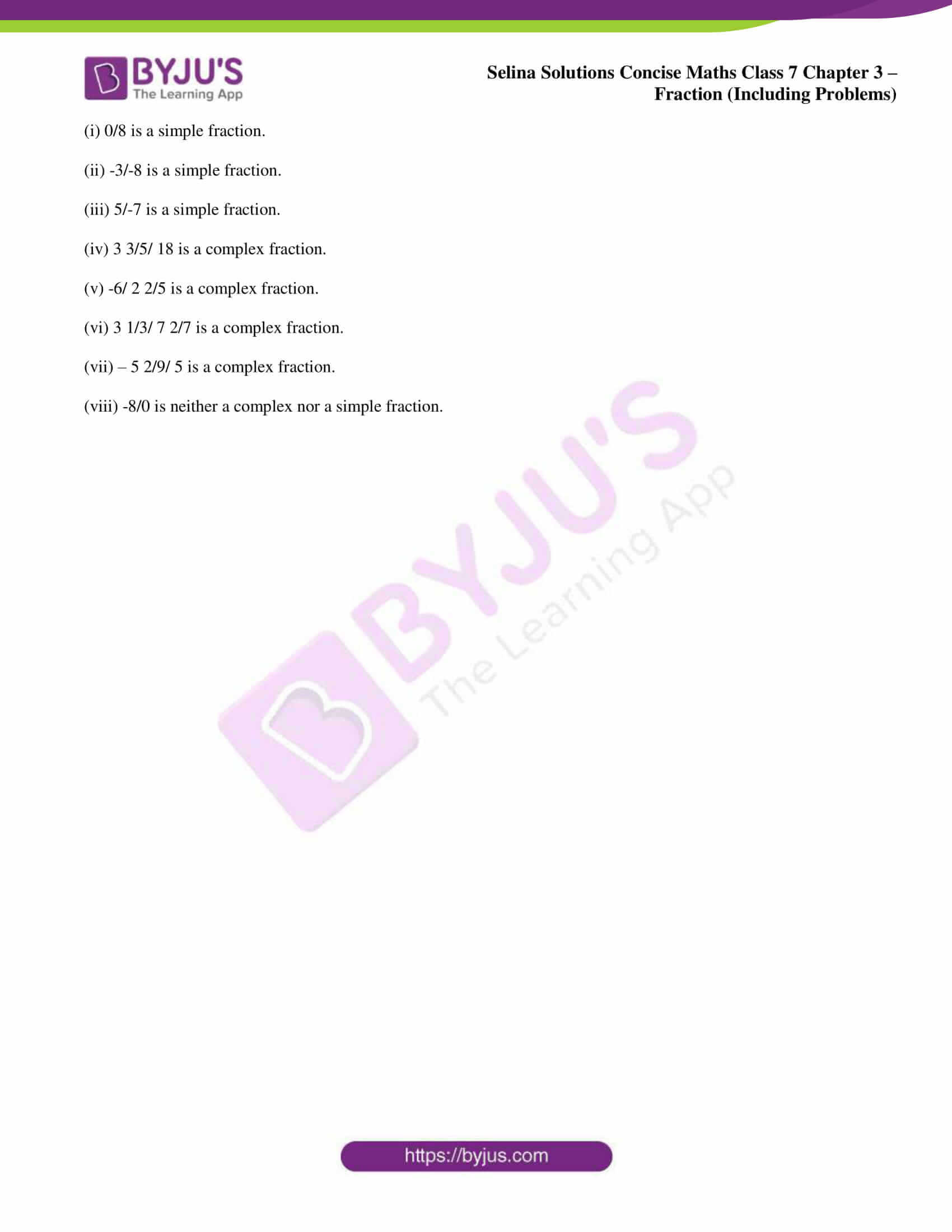# Selina Solutions Concise Maths Class 7 Chapter 3: Fraction (Including Problems) Exercise 3A

Selina Solutions Concise Maths Class 7 Chapter 3 Fraction (Including Problems) Exercise 3A has answers designed by experts at BYJU’S, who have vast experience in the education industry. This exercise basically helps students gain a better idea about the classification of the given set of fractions. The methods to be followed in reducing a given fraction to its lowest term is explained clearly in the solutions PDF. Students can download Selina Solutions Concise Maths Class 7 Chapter 3 Fraction (Including Problems) Exercise 3A PDF from the links, which are provided here.

## Selina Solutions Concise Maths Class 7 Chapter 3: Fraction (Including Problems) Exercise 3A Download PDF### Access Selina Solutions Concise Maths Class 7 Chapter 3: Fraction (Including Problems) Exercise 3A

1. Classify each fraction given below as decimal or vulgar fraction, proper or improper fraction and mixed fraction:

(i) 3/5

(ii) 11/10

(iii) 13/20

(iv) 18/7

(v) 3 2/9

Solution:

(i) 3/5 is a vulgar and proper fraction.

(ii) 11/10 is a decimal and improper fraction.

(iii) 13/20 is a decimal and proper fraction.

(iv) 18/7 is a vulgar and improper fraction.

(v) 3 2/9 is a mixed fraction.

2. Express the following improper fractions as mixed fractions:

(i) 18/5

(ii) 7/4

(iii) 25/6

(iv) 38/5

(v) 22/5

Solution:

(i) 18/5 can be expressed as mixed fractions as 3 3/5.

(ii) 7/4 can be expressed as mixed fractions as 1 3/4.

(iii) 25/6 can be expressed as mixed fractions as 4 1/6.

(iv) 38/5 can be expressed as mixed fractions as 7 3/5.

(v) 22/5 can be expressed as mixed fractions as 4 2/5.

3. Express the following mixed fractions as improper fractions:

(i) 2 4/9

(ii) 7 5/13

(iii) 3 1/4

(iv) 2 5/48

(v) 12 7/11

Solution:

(i) 2 4/9

It can be written as

= (2 × 9 + 4)/ 9

By further calculation

= (18 + 4)/ 9

= 22/9

(ii) 7 5/13

It can be written as

= (7 × 13 + 5)/ 13

By further calculation

= (91 + 5)/ 13

= 96/13

(iii) 3 1/4

It can be written as

= (3 × 4 + 1)/ 4

By further calculation

= (12 + 1)/ 4

= 13/4

(iv) 2 5/48

It can be written as

= (2 × 48 + 5)/ 48

By further calculation

= (96 + 5)/ 48

= 101/48

(v) 12 7/11

It can be written as

= (12 × 11 + 7)/ 11

By further calculation

= (132 + 7)/ 11

= 139/11

4. Reduce the given fractions to lowest terms:

(i) 8/18

(ii) 27/36

(iii) 18/42

(iv) 35/75

(v) 18/45

Solution:

(i) 8/18

Here the HCF of 8 and 18 is 2

So by dividing numerator and denominator by 2

= (8 ÷ 2)/ (18 ÷ 2)

We get

= 4/9

(ii) 27/36

Here the HCF of 27 and 36 is 9

So by dividing numerator and denominator by 9

= (27 ÷ 9)/ (36 ÷ 9)

We get

= 3/4

(iii) 18/42

Here the HCF of 18 and 42 is 6

So by dividing both numerator and denominator by 6

= (18 ÷ 6)/ (42 ÷ 6)

We get

= 3/7

(iv) 35/75

Here the HCF of 35 and 75 is 5

So by dividing both numerator and denominator by 5

= (35 ÷ 5)/ (75 ÷ 5)

We get

= 7/15

(v) 18/45

Here the HCF of 18 and 45 is 9

So by dividing both numerator and denominator by 9

= (18 ÷ 9)/ (45 ÷ 9)

We get

= 2/5

5. State true or false:

(i) 30/40 and 12/16 are equivalent fractions.

(ii) 10/25 and 25/10 are equivalent fractions.

(iii) 35/49, 20/28, 45/63 and 100/140 are equivalent fractions.

Solution:

(i) True.

Here 30/40 = 3/4 and 12/16 = 3/4

(ii) False.

Here 10/25 = 2/5 and 25/10 = 5/2

(iii) True.

35/49 = 5/7, 20/28 = 5/7, 45/63 = 5/7 and 100/140 = 5/7 where all are equal.

6. Distinguish each of the fractions, given below, as a simple fraction or a complex fraction:

(i) 0/8

(ii) -3/-8

(iii) 5/-7

(iv) 3 3/5/ 18

(v) -6/ 2 2/5

(vi) 3 1/3/ 7 2/7

(vii) – 5 2/9/ 5

(viii) -8/0

Solution:

(i) 0/8 is a simple fraction.

(ii) -3/-8 is a simple fraction.

(iii) 5/-7 is a simple fraction.

(iv) 3 3/5/ 18 is a complex fraction.

(v) -6/ 2 2/5 is a complex fraction.

(vi) 3 1/3/ 7 2/7 is a complex fraction.

(vii) – 5 2/9/ 5 is a complex fraction.

(viii) -8/0 is neither a complex nor a simple fraction.